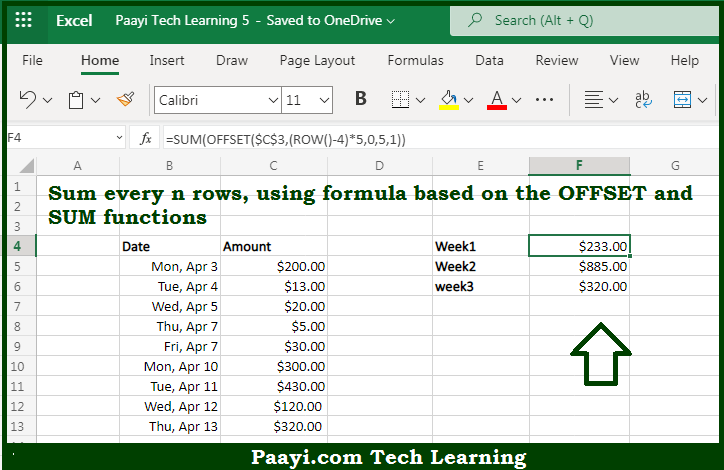# Learn How to SUM Every n Rows in Microsoft Excel

Written by | 0 Comments | 473 Views

In this article, you will learn how to COUNT various things in Microsoft Excel using a single/combination(s) of functions. You will also know how to SUM Every n Rows and see the generic formula.

SUM Every n Rows in Microsoft Excel

The main purpose of this formula is to sum every n row. Here we will learn how to sum every n row provided in the workbook in Microsoft Excel. That implies, with the help of a formula based on the SUM and OFFSET function you can sum every n row provided. So, with the help of this formula, you can able to sum every n row in the workbook in Microsoft Excel.

General Formula to SUM Every n Rows

=SUM(OFFSET(A1,(ROW()-offset)*n,0,n,1))

The Explanation for the SUM Every n RowsSo we know that with the help of the given formula above you can able sum every n row. Here we will learn how to sum every n row provided in the workbook in Microsoft Excel. As we know that the there are five data rows each week is given in the example. And we want to sum every five rows. To build a range that corresponds to the right five rows in each week, we are using the OFFSET function. The OFFSET function finally returns a range to SUM, and SUM returns the sum of all amounts in that range. So, with the help of this formula, you can able to sum every n row provided in the workbook in Microsoft Excel.